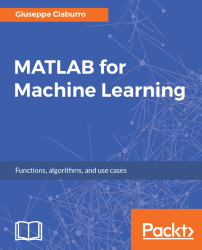•#### MATLAB for Machine Learning#### Overview of this book

MATLAB is the language of choice for many researchers and mathematics experts for machine learning. This book will help you build a foundation in machine learning using MATLAB for beginners. You’ll start by getting your system ready with t he MATLAB environment for machine learning and you’ll see how to easily interact with the Matlab workspace. We’ll then move on to data cleansing, mining and analyzing various data types in machine learning and you’ll see how to display data values on a plot. Next, you’ll get to know about the different types of regression techniques and how to apply them to your data using the MATLAB functions. You’ll understand the basic concepts of neural networks and perform data fitting, pattern recognition, and clustering analysis. Finally, you’ll explore feature selection and extraction techniques for dimensionality reduction for performance improvement. At the end of the book, you will learn to put it all together into real-world cases covering major machine learning algorithms and be comfortable in performing machine learning with MATLAB.
Title PageCreditsForewordwww.PacktPub.comCustomer FeedbackPrefaceFree Chapter
Getting Started with MATLAB Machine LearningImporting and Organizing Data in MATLABFrom Data to Knowledge DiscoveryFinding Relationships between Variables - Regression TechniquesPattern Recognition through Classification AlgorithmsIdentifying Groups of Data Using Clustering MethodsSimulation of Human Thinking - Artificial Neural NetworksImproving the Performance of the Machine Learning Model - Dimensionality ReductionMachine Learning in Practice## Data organization

So far, for data organization, we have mostly used standard arrays that represent useful data structures for storing a large number of objects, but all of the same type, such as a matrix of numbers or characters. However, such arrays cannot be used if you want to memorize both numbers and strings in the same object. This is a problem that can be solved by so-called cell arrays, structure arrays, and more generally all those structures that the MATLAB programming environment provides us.

### Cell array

A cell array is a datatype that has indexed data containers called cells. Each cell can contain any type of data; cell arrays can contain, for example, text strings, combinations of text and numbers, or numeric arrays of different sizes.

To create a cell array, we can simply use the cell array construction operator, that is, the `{}` operator (braces), we can see as (name and age of my family members):

```>> MyFamily = {'Luigi', 'Simone', 'Tiziana'; 13, 11, 43}
MyFamily =
2×3 cell...```